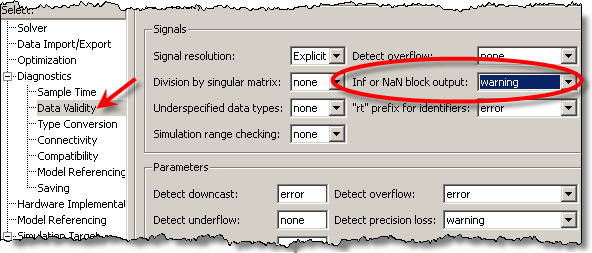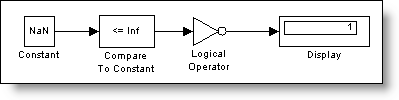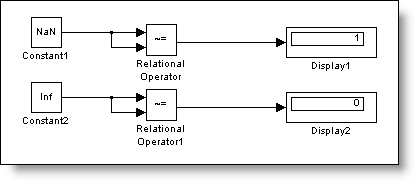# How do I test for NaN in my model?

This week I want to pose a brainteaser that my colleague Jeff had to solve this week.  Imagine you need to detect the condition where a signal value might become NaN (Not A Number).  How do you test for that in Simulink?

What is a NaN?

NaN means Not-A-Number.  In general math terms, this is the result for special cases, which are undefined.  In general computing terms, operations return NaN for invalid inputs.  For example:

>> Inf*0
ans =
NaN
>> 0/0
ans =
NaN
>> Inf/Inf
ans =
NaN
>> Inf-Inf
ans =
NaN

If you are writing code and need to protect against invalid inputs, detecting this could be important.

ISNAN?

In MATLAB we have the function ISNAN.  ISNAN is a MATLAB function, which we could call from Simulink.

>> x = 0/0
x =
NaN
>> isnan(x)
ans =
1

In some ways, that feels like cheating.  If we were developing this algorithm to run on an embedded system, MATLAB will not be available.

First try, Diagnostics

The first idea I had was to look at the diagnostics.  I remembered seeing a warning about NaNs once during a simulation, so there might be a way to turn on a diagnostic to detect this.We found the diagnostic in the Data Validity page of the Configuration Parameters.  This diagnostic will check for Inf or NaN signals in a block output, but that doesn’t help if we want the model to do something reactive with that information.  This diagnostic is purely informative.

NaN has a specific bit pattern

What about testing the bit pattern?  Using MATLAB I can see what that pattern is in HEX:

>> format hex
>> NaN
ans =
fff8000000000000

We did try the bit operations, but ran into some difficulties.  NaN is a floating-point number, and all the bit processing blocks are built for working with integers.  In this case, working with a floating-point number would require some contortions to get the bit pattern.  Once we had it, we could use the other Bitwise Operator block to test for the pattern we were looking for, but we didn’t get that working before we had another realization.

Comparison with NaN is different from all other numbers

That was the “Eureka!” moment: NaN has special properties!  Math operations performed with NaN result in a NaN  (x+NaN = NaN).  Comparisons to a NaN return false.  For example:

>> NaN<1
ans =
0
>> NaN<=Inf
ans =
0

Comparison with Inf is pretty interesting because we couldn’t think of any other numbers that would return FALSE for X<=Inf, except NaN.  Even if X=Inf, this should return TRUE.  In Simulink, the test model looked like this:Using the logical operation, NOT, we can pass any signal through these blocks and make our own ISNAN block.  Another interesting property of NaN is that it is the ONLY number not equal to itself.  An alternative model, arguably more streamlined, uses just the Relational Operator to test the NOT Equals condition and compares the signal to itself.All numbers EXCEPT NaN should return FALSE for this test.  We double-checked it by sending infinity through the same construct.